NEET  >  Test: Electric Flux & Electric Dipole (NCERT)

# Test: Electric Flux & Electric Dipole (NCERT)

Test Description

## 10 Questions MCQ Test Physics Class 12 | Test: Electric Flux & Electric Dipole (NCERT)

Test: Electric Flux & Electric Dipole (NCERT) for NEET 2023 is part of Physics Class 12 preparation. The Test: Electric Flux & Electric Dipole (NCERT) questions and answers have been prepared according to the NEET exam syllabus.The Test: Electric Flux & Electric Dipole (NCERT) MCQs are made for NEET 2023 Exam. Find important definitions, questions, notes, meanings, examples, exercises, MCQs and online tests for Test: Electric Flux & Electric Dipole (NCERT) below.
Solutions of Test: Electric Flux & Electric Dipole (NCERT) questions in English are available as part of our Physics Class 12 for NEET & Test: Electric Flux & Electric Dipole (NCERT) solutions in Hindi for Physics Class 12 course. Download more important topics, notes, lectures and mock test series for NEET Exam by signing up for free. Attempt Test: Electric Flux & Electric Dipole (NCERT) | 10 questions in 10 minutes | Mock test for NEET preparation | Free important questions MCQ to study Physics Class 12 for NEET Exam | Download free PDF with solutions
 1 Crore+ students have signed up on EduRev. Have you?
Test: Electric Flux & Electric Dipole (NCERT) - Question 1

### The SI unit of electric flux is

Detailed Solution for Test: Electric Flux & Electric Dipole (NCERT) - Question 1

As electric flux, φ = E.Δs
∴ unit of φ is N C-1 m2

Test: Electric Flux & Electric Dipole (NCERT) - Question 2

### The dimensional formula of electric flux is

Detailed Solution for Test: Electric Flux & Electric Dipole (NCERT) - Question 2

Electric flux, φ=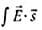The dimension of φ = Dimension of E x dimension of s
[φ] = [M1L1T-2][AT]-1[L2] = [M1L3T-3A-1]

Test: Electric Flux & Electric Dipole (NCERT) - Question 3

### A circular plane sheet of radius 10 cm is placed in a uniform electric field of 5 x 105N C-1, making an angle of 600 with the field. The electric flux through the sheet is

Detailed Solution for Test: Electric Flux & Electric Dipole (NCERT) - Question 3

Here, r = 10 cm 0.1 m, E = 5 x 105 N C-1
As the angle between the plane sheet and the electric field is 600, angle made by the normal to the plane sheet and the electric field is θ = 900 - 600 = 300
φE = ES cos θ = E x πr2 cos θ
= 5 x 105 x 3.14 x (0.1)2 cos 300 = 1.36 x 104 N m2C-1

Test: Electric Flux & Electric Dipole (NCERT) - Question 4

A uniform electric field E = 2 x 103 N C-1 is acting along the positive x-axis. The flux of this field through a square of 10cm on a side whose plane is paralled to the yz plane is

Detailed Solution for Test: Electric Flux & Electric Dipole (NCERT) - Question 4

Here, E = 2 x 103 N C-1 is along + x-axis
Surface area, s = (10 cm)2 = 10x 10-4 m2 = 10-2 m2
When plane is parallel to yz plane, θ = 0°
So φ = E s cosθ = 2 x 103 x 10-2 cos 0° = 20N C-1 m2

Test: Electric Flux & Electric Dipole (NCERT) - Question 5

In the question number 66, the flux through the same square if the line normal to its plane makes a 60° angle with the x-axis is

Detailed Solution for Test: Electric Flux & Electric Dipole (NCERT) - Question 5

When normal to the plane makes an angle of 60° with x-axis, then θ = 60°
φ = Es cosθ = 2 x 103 x 10-2 cos 60° = 10 N C-1N C-1m2

Test: Electric Flux & Electric Dipole (NCERT) - Question 6

Which of the following statements about dipole moment is not true?

Detailed Solution for Test: Electric Flux & Electric Dipole (NCERT) - Question 6

Dipole moment is a vector quantity and has magnitude of 2qa and it is in the direction of the dipole axis from -q to q.

Test: Electric Flux & Electric Dipole (NCERT) - Question 7

The unit of electric dipole moment is

Detailed Solution for Test: Electric Flux & Electric Dipole (NCERT) - Question 7

The unit of electric dipole moment is: 2ql =  C − m = Debye

Test: Electric Flux & Electric Dipole (NCERT) - Question 8

Two charges + 20 μC and -20 μC are placed 10 mm apart. The electric field at point P, on the axis of the dipole 10 cm away from  its centre O on the side of the positive charge is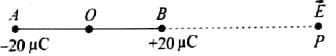Detailed Solution for Test: Electric Flux & Electric Dipole (NCERT) - Question 8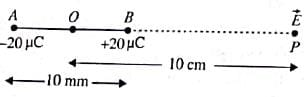Here, q = ±20μC = ±20 x 10-6C, 2a = 10 mm = 10 x 10-3m
r = OP = 10 cm = 10 x 10-2 m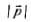= q x 2a = 20 x 10-6 x 10 x 10-3 m = 2 x 10-7 m
The electric field along BP,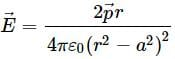As a < < r,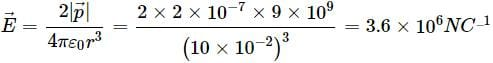Test: Electric Flux & Electric Dipole (NCERT) - Question 9

Consider a region inside which, there are various types of charges but the total charge is zero. At points outside the region

Detailed Solution for Test: Electric Flux & Electric Dipole (NCERT) - Question 9

When there are various types of charges in a region, but the total charge is zero, the region can be supposed to contain a number of electric dipoles. Therefore, at points outside the region, the dominant electric field ∝ (1/r3) for large r.

Test: Electric Flux & Electric Dipole (NCERT) - Question 10

Two point charges of 1μC and -1μC are separated by a distance of 100 Å. A point P is at a distance of 10 cm from the midpoint and on the perpendicular bisector of the line joining the two charges. The electric field at P will be

Detailed Solution for Test: Electric Flux & Electric Dipole (NCERT) - Question 10

The Point lies on equatorial line of a short dipole,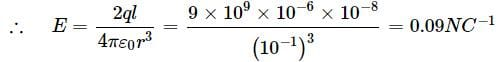## Physics Class 12

157 videos|452 docs|213 tests
 Use Code STAYHOME200 and get INR 200 additional OFF Use Coupon Code
Information about Test: Electric Flux & Electric Dipole (NCERT) Page
In this test you can find the Exam questions for Test: Electric Flux & Electric Dipole (NCERT) solved & explained in the simplest way possible. Besides giving Questions and answers for Test: Electric Flux & Electric Dipole (NCERT), EduRev gives you an ample number of Online tests for practice

## Physics Class 12

157 videos|452 docs|213 tests

### How to Prepare for NEET

Read our guide to prepare for NEET which is created by Toppers & the best Teachers